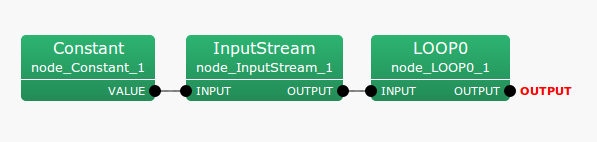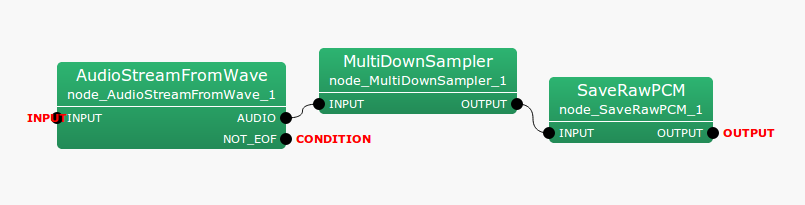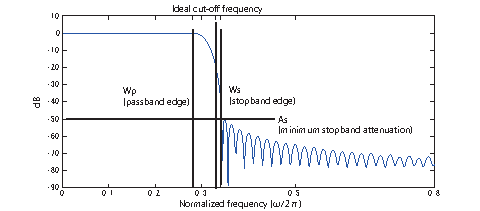## 6.7.20 MultiDownSampler

### 6.7.20.1 Outline of the node

This node performs downsampling of input signals and outputs their results. The window method is used for low-pass filters and its window function is Kaiser window.

### 6.7.20.2 Necessary files

No files are required.

### 6.7.20.3 Usage

When to use

This node is used when the sampling frequency of input signals is not 16 kHz. For the HARK nodes, the default sampling frequency is 16kHz. If, for example, the input signals are 48 kHz, downsampling is required to reduce the sampling frequency to 16 kHz. Note 1 (Range of ADVANCE): To make processing more convenient, it is necessary to limit the parameter settings of input nodes that are connected before nodes such as AudioStreamFromMic and AudioStreamFromWave . Differences in the parameters LENGTH and ADVANCE: OVERLAP = LENGTH - ADVANCE must be sufficiently large. More concretely, the differences must be greater than the low-pass filter length $N$ of this node. Values over 120 are sufficient for the default setting of this node and therefore no problems occur if ADVANCE is more than a quarter of LENGTH. Moreover, it is necessary to satisfy the requirements below. Note 2 (Setting of ADVANCE): The ADVANCE value of this node must be SAMPLING_RATE_IN / SAMPLING_RATE_OUT times as great as the ADVANCE value of the node connected afterward (e.g. GHDSS ). Since this is a specification, its operation is not guaranteed with values other than those above. For example, if ADVANCE = 160 and of SAMPLING_RATE_IN / SAMPLING_RATE_OUT is 3 for the node connected later, it is necessary to set the ADVANCE of this node and that connected before to 480. Note 3 (Requirements for the LENGTH value of the node connected before this node): The LENGTH value of the node connected before this node (e.g. AudioStreamFromMic ) must be SAMPLING_RATE_IN / SAMPLING_RATE_OUT times as great as the ADVANCE value of the node connected afterward (e.g. GHDSS ). For example, if SAMPLING_RATE_IN / SAMPLING_RATE_OUT is 3, and LENGTH is 512 and ADVANCE is 160 for GHDSS , then LENGTH should be 1536 and ADVANCE should be 480 for AudioStreamFromMic .

Typical connection

Examples of typical connections are shown below. This network file reads Wave file inputs, performs downsampling and saves files as Raw files. Wave file input is achieved by connecting Constant , InputStream and AudioStreamFromMic . This is followed by downsampling with MultiDownSampler , with output waveforms saved in SaveRawPCM .Figure 6.116: Example of a connection of MultiDownSampler : Main networkFigure 6.117: Example of a connection of MultiDownSampler : Iteration (LOOP0) Network

### 6.7.20.4 Input-output and property of the node

Input

INPUT

: Matrix<float> type. Multichannel speech waveform data (time domain waveform).

Output

OUTPUT

: Matrix<float> type. The multichannel speech waveform data for which downsampling is performed (time domain waveform).

Table 6.119: Parameter list of MultiDownSampler
 Parameter name Type Default Value Unit Description ADVANCE 480 [pt] Frame shift length for every iteration in INPUT signals. Since special setting is required, see the parameter description. SAMPLING_RATE_IN 48000 [Hz] Sampling frequency of INPUT signals. SAMPLING_RATE_OUT 16000 [Hz] Sampling frequency of OUTPUT signals. Wp 0.28 [$\frac{\omega }{2\pi }$] Low-pass filter pass band end. Designate normalized frequency [0.0 - 1.0] with INPUT as reference. Ws 0.34 [$\frac{\omega }{2\pi }$] Low-pass filter stopband end. Designate normalized frequency [0.0 - 1.0] with INPUT as reference. As 50 [dB] Minimum attenuation in stopband.

Parameter

Low-pass filters, frequency characteristics of a Kaiser window, are mostly set for the parameters. Figure 6.118 shows the relationships between symbols and filter properties. Note the correspondence when reading them.

: int type. The default value is 480. For processing frames for speech waveforms, designate the shift width on waveforms in sampling numbers. Here, use the values of the nodes connected prior to INPUT. Note: This value must be SAMPLING_RATE_IN / SAMPLING_RATE_OUT times as great as the ADVANCE value set for after OUTPUT.

SAMPLING_RATE_IN

: int type. The default value is 48000. Designate sampling frequency for input waveforms.

SAMPLING_RATE_OUT

: int type. The default value is 16000. Designate sampling frequency for output waveforms. Values that can be used are 1 / integer of SAMPLING_RATE_IN.

Wp

: float type. The default value is 0.28. Designate the low-pass filter pass band end frequency by values of normalized frequency [0.0 - 1.0] with INPUT as reference. When the sampling frequency of inputs is 48000 [Hz] and this value is set to 0.48, gains of low-pass filter begin to decrease from around $48000 * 0.28 = 13440$ [Hz].

Ws

: float type. The default value is 0.34. Designate the low-pass filter stopband end frequency by values of normalized frequency [0.0 - 1.0] with INPUT as reference. When the sampling frequency of inputs is 48000[Hz] and this value is set to 0.38, gains of low-pass tilter begin to be stable from around $48000 * 0.34 = 16320$ [Hz].

As

: float type. The default value is 50. Designate the value indicating the minimum attenuation in stopband in [dB]. When using the default value, the gain of the stopband is around -50 [dB], with the passing band as 0.

When Wp, Ws and As are set at their default values, Wp and Ws will be around the cutoff frequency $Ws$. For example, the accuracy of the frequency response characteristic of Kaiser window will improve. However, the dimensions of the low-pass filter and the processing time will be increased. This relationship is considered a trade off.Figure 6.118: Filter properties of default settings.

### 6.7.20.5 Details of the node

MultiDownSampler is the node that uses the low-pass filter for band limiting, using the Kaiser window for multichannel signals and downsampling. This node downsamples ${SAMPLING\_ RATE\_ OUT} / {SAMPLING\_ RATE\_ IN}$ after creating / executing an FIR low-pass filter by synthesizing 1) a Kaiser window and 2) ideal low-pass responses.

FIR filter: Filtering with a finite impulse response $h(n)$ is performed based on the equation

 $\displaystyle s_{{out}}(t) = \sum _{i = 0}^{N} h(n) s_{{in}}(t-n).$ (165)

Here, $s_{{out}}(t)$ indicates output signals and $s_{{in}}(t)$ indicates input signals. For multichannel signals, the signals of each channel are filtered independently. The same finite impulse response $h(n)$ is used here. Ideal low-pass response: The ideal low-pass response with a cutoff frequency of $\omega _ c$ is obtained using the equation

 $\displaystyle H_ i(e^{j\omega }) = \left\{ \begin{array}{cc}1, & |\omega |< \omega _ c \\ 0, & {otherwise} \end{array} \right.$ (166)

This impulse response is expressed as

 $\displaystyle h_ i(n) = \frac{\omega _ c}{\pi } \left( \frac{sin(\omega n)}{\omega n} \right),~ ~ -\infty \leq n \leq \infty$ (167)

This impulse response does not satisfy the acausal and bounded input-bounded output (BIBO) stability conditions. It is therefore necessary to cut off the impulse response in the middle to obtain the FIR filter from this ideal filter.

 $\displaystyle h(n)= \left\{ \begin{array}{ll} h_ i(n), & |n|\leq \frac{N}{2} \\ 0, & {otherwise} \end{array} \right.$ (168)

Here, $N$ indicates a dimension of the filter. In this filter, cutoff of the impulse response results in ripples in the pass band and stopband. Moreover, the minimum attenuation in stopband $As$ remains around 21dB and sufficient attenuation is not obtained.

Low-pass filter by the window method with Kaiser window: To improve the properties of the above cutoff method, an impulse response, in which the ideal impulse response $h_ i(n)$ is multiplied by the window function $v(n)$, is used instead.

 $\displaystyle h(n)= h_ i(n) v(n)$ (169)

Here, the low-pass filter is designed with the Kaiser window. The Kaiser window is defined by the equation

 $\displaystyle v(n)= \left\{ \begin{array}{ll} \frac{ I_0 \left( \beta \sqrt {1 - (n N / 2)^2} \right)}{I_0(\beta )}, & -\frac{N}{2} \leq n \leq \frac{N}{2} \\ 0, & {otherwise} \end{array} \right.$ (170)

Here, $\beta$ indicates the parameter determining the shape of the window and $I_0(x)$ indicates the modified Bessel function of 0th order. The Kaiser window is obtained using the equation

 $\displaystyle I_0(x)= 1 + \sum _{k=1}^{\infty } \left( \frac{(0.5 x)^ k}{k!} \right)$ (171)

The parameter $\beta$ is determined by the attenuation obtained by the low-pass filter. Here, it is determined by the index,

 $\displaystyle \beta = \left\{ \begin{array}{ll} 0.1102 (As - 8.7) & As > 50, \\ 0,5842 (As - 21)^{0.4} + 0.07886 (As - 21) & 21 < As < 50, \\ 0 & As < 21 \end{array} \right.$ (172)

If the cutoff frequency $\omega _ c$ and the filter order have been determined, the low-pass filter can be determined by the window method. The filter order $N$ can be estimated with the minimum attenuation in stopband As and the transition region $\Delta f = (Ws - Wp) / (2\pi )$ as,

 $\displaystyle N \approx \frac{As - 7.95}{14.36 \Delta f}$ (173)

Moreover, the cutoff frequency $\omega _ c$ is set to $0.5 (Wp + Ws)$. Downsampling: Downsampling is realized by thinning the sample points of SAMPLING_RATE_IN / SAMPLING_RATE_OUT from the signals that pass the low-pass filter. For example, $48000 / 16000 = 3$ in the default setting; therefore, input samples taken once every three times will be output samples.

### 6.7.20.6 References:

(1) Author: Translated by P. Vaidyanathan: Akinori Nishihara, Eiji Watanabe, Toshiyuki Yoshida, Nobuhiko Sugino: "Multirate signal processing and filter bank", Science and technology publication, 2001.